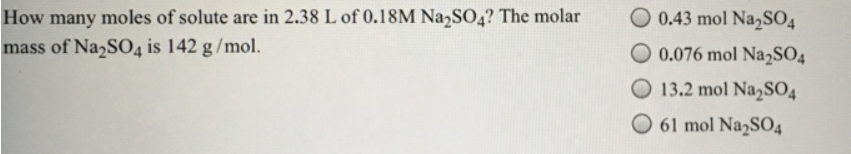# Problem: How many moles of solute are in 2.38 L of 0.18M Na2SO4? The molar mass of Na2SO4 is 142 g/mol. (a) 0.43 mol Na2SO4 (b) 0.076 mol Na2SO4 (c) 13.2 mol Na2SO4 (d) 61 mol Na2SO4

###### FREE Expert Solution
79% (267 ratings)###### Problem Details

How many moles of solute are in 2.38 L of 0.18M Na2SO4? The molar mass of Na2SO4 is 142 g/mol.

(a) 0.43 mol Na2SO4

(b) 0.076 mol Na2SO4

(c) 13.2 mol Na2SO4

(d) 61 mol Na2SO4Frequently Asked Questions

What scientific concept do you need to know in order to solve this problem?

Our tutors have indicated that to solve this problem you will need to apply the Molarity concept. You can view video lessons to learn Molarity. Or if you need more Molarity practice, you can also practice Molarity practice problems.

What professor is this problem relevant for?

Based on our data, we think this problem is relevant for Professor Keister's class at UB.